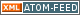www.LinuxHowtos.org howtos, tips&tricks and tutorials for linuxfrom small one page howto to huge articles all in one place

search text in:

Poll
Which kernel version do you use?

poll results

using iotop to find disk usage hogs

using iotop to find disk usage hogs

words:

887

views:

138595

userrating:

average rating: 1.8 (88 votes) (1=very good 6=terrible)

May 25th. 2007:
Words

486

Views

241589

Workaround and fixes for the current Core Dump Handling vulnerability affected kernels

Workaround and fixes for the current Core Dump Handling vulnerability affected kernels

words:

161

views:

123749

userrating:

average rating: 1.3 (28 votes) (1=very good 6=terrible)

April, 26th. 2006:

You are here: manpages

# MALLOC_INFO

Section: Linux Programmer's Manual (3)
Updated: 2017-09-15

## NAME

malloc_info - export malloc state to a stream

## SYNOPSIS

```#include <malloc.h>

int malloc_info(int options, FILE *stream);
```

## DESCRIPTION

The malloc_info() function exports an XML string that describes the current state of the memory-allocation implementation in the caller. The string is printed on the file stream stream. The exported string includes information about all arenas (see malloc(3)).

As currently implemented, options must be zero.

## RETURN VALUE

On success, malloc_info() returns 0; on error, it returns -1, with errno set to indicate the cause.

## ERRORS

EINVAL
options was nonzero.

## VERSIONS

malloc_info() was added to glibc in version 2.10.

## ATTRIBUTES

For an explanation of the terms used in this section, see attributes(7).
 Interface Attribute Value malloc_info() Thread safety MT-Safe

## CONFORMING TO

This function is a GNU extension.

## NOTES

The memory-allocation information is provided as an XML string (rather than a C structure) because the information may change over time (according to changes in the underlying implementation). The output XML string includes a version field.

The open_memstream(3) function can be used to send the output of malloc_info() directly into a buffer in memory, rather than to a file.

The malloc_info() function is designed to address deficiencies in malloc_stats(3) and mallinfo(3).

## EXAMPLE

The program below takes up to four command-line arguments, of which the first three are mandatory. The first argument specifies the number of threads that the program should create. All of the threads, including the main thread, allocate the number of blocks of memory specified by the second argument. The third argument controls the size of the blocks to be allocated. The main thread creates blocks of this size, the second thread created by the program allocates blocks of twice this size, the third thread allocates blocks of three times this size, and so on.

The program calls malloc_info() twice to display the memory-allocation state. The first call takes place before any threads are created or memory allocated. The second call is performed after all threads have allocated memory.

In the following example, the command-line arguments specify the creation of one additional thread, and both the main thread and the additional thread allocate 10000 blocks of memory. After the blocks of memory have been allocated, malloc_info() shows the state of two allocation arenas.

\$ getconf GNU_LIBC_VERSION glibc 2.13 \$ ./a.out 1 10000 100 ============ Before allocating blocks ============ <malloc version="1"> <heap nr="0"> <sizes> </sizes> <total type="fast" count="0" size="0"/> <total type="rest" count="0" size="0"/> <system type="current" size="135168"/> <system type="max" size="135168"/> <aspace type="total" size="135168"/> <aspace type="mprotect" size="135168"/> </heap> <total type="fast" count="0" size="0"/> <total type="rest" count="0" size="0"/> <system type="current" size="135168"/> <system type="max" size="135168"/> <aspace type="total" size="135168"/> <aspace type="mprotect" size="135168"/> </malloc>

============ After allocating blocks ============ <malloc version="1"> <heap nr="0"> <sizes> </sizes> <total type="fast" count="0" size="0"/> <total type="rest" count="0" size="0"/> <system type="current" size="1081344"/> <system type="max" size="1081344"/> <aspace type="total" size="1081344"/> <aspace type="mprotect" size="1081344"/> </heap> <heap nr="1"> <sizes> </sizes> <total type="fast" count="0" size="0"/> <total type="rest" count="0" size="0"/> <system type="current" size="1032192"/> <system type="max" size="1032192"/> <aspace type="total" size="1032192"/> <aspace type="mprotect" size="1032192"/> </heap> <total type="fast" count="0" size="0"/> <total type="rest" count="0" size="0"/> <system type="current" size="2113536"/> <system type="max" size="2113536"/> <aspace type="total" size="2113536"/> <aspace type="mprotect" size="2113536"/> </malloc>

### Program source

#include <unistd.h> #include <stdlib.h> #include <pthread.h> #include <malloc.h> #include <errno.h>

static size_t blockSize; static int numThreads, numBlocks;

#define errExit(msg) do { perror(msg); exit(EXIT_FAILURE); \
} while (0)

static void * thread_func(void *arg) {
int j;
int tn = (int) arg;

/* The multiplier aq(2 + tn)aq ensures that each thread (including
the main thread) allocates a different amount of memory */

for (j = 0; j < numBlocks; j++)
if (malloc(blockSize * (2 + tn)) == NULL)

sleep(100);         /* Sleep until main thread terminates */
return NULL; }

int main(int argc, char *argv[]) {
int j, tn, sleepTime;

if (argc < 4) {
fprintf(stderr,
argv);
exit(EXIT_FAILURE);
}

numBlocks = atoi(argv);
blockSize = atoi(argv);
sleepTime = (argc > 4) ? atoi(argv) : 0;

if (thr == NULL)
errExit("calloc");

printf("============ Before allocating blocks ============\n");
malloc_info(0, stdout);

/* Create threads that allocate different amounts of memory */

for (tn = 0; tn < numThreads; tn++) {
(void *) tn);
if (errno != 0)

/* If we add a sleep interval after the start-up of each
mutexes, and therefore additional arenas wonaqt be
allocated (see malloc(3)). */

if (sleepTime > 0)
sleep(sleepTime);
}

/* The main thread also allocates some memory */

for (j = 0; j < numBlocks; j++)
if (malloc(blockSize) == NULL)
errExit("malloc");

sleep(2);           /* Give all threads a chance to
complete allocations */

printf("\n============ After allocating blocks ============\n");
malloc_info(0, stdout);

exit(EXIT_SUCCESS); }

mallinfo(3), malloc(3), malloc_stats(3), mallopt(3), open_memstream(3)

## Index

NAME
SYNOPSIS
DESCRIPTION
RETURN VALUE
ERRORS
VERSIONS
ATTRIBUTES
CONFORMING TO
NOTES
EXAMPLE
Program source
COLOPHON||- Powered by- Running on-
Copyright 2004-2020 Sascha Nitsch Unternehmensberatung GmbH::- Level Triple-A Conformance to Web Content Accessibility Guidelines 1.0 -
- Copyright and legal notices -